# 条件生成对抗模型生成数字图片

+关注继续查看

## 第一步：import 我们需要的数据库

%matplotlib inline

from __future__ import print_function, division

import numpy as np
import pandas as pd
import matplotlib.pyplot as plt
import matplotlib.image as mpimg
import matplotlib.cm as cm

import seaborn as sns
sns.set_style('white')

from keras.layers import Input, Dense, Reshape, Flatten, Dropout, multiply
from keras.layers import BatchNormalization, Activation, Embedding, ZeroPadding2D
from keras.layers.convolutional import UpSampling2D, Conv2D
from keras.models import Sequential, Model
from keras.optimizers import Adam, SGD

## 第二步：数据预处理

2.1 数据加载函数

def load_data():
print(data.files)
x_train = data['x_train']
y_train = data['y_train']
x_test = data['x_test']
y_test = data['y_test']

x_train = (x_train.astype(np.float32) - 127.5) / 127.5
x_train = np.expand_dims(x_train, axis=3)
y_train = y_train.reshape(-1, 1)

return (x_train, y_train), (x_test, y_test)

(x_train, y_train), (x_test, y_test)=load_data()

2.2 数据查看

2.2.1 查看原始数据的纬度

print(x_train.shape)

print(x_test.shape)

2.2.2 查看标签的数量

print(y_train.shape)

print(y_test.shape)

2.2.3 查看所有的标签种类

np.unique(y_train)

np.unique(y_test)

2.3数据可视化

plt.figure(figsize=(15, 9))
for i in range(50):
random_selection = np.random.randint(0, 500)
plt.subplot(5, 10, 1+i)
plt.title(y_train[random_selection])
plt.imshow(x_train[random_selection][:,:,0], cmap=cm.gray)

2.4 查看数据是否平衡

sns.distplot(y_train, kde=False, bins=10)

## 第三步：构建模型3.3 定义网络

3.3.1先定义一些常量

# 输入图片数据的维度
img_shape = (28, 28, 1)

# 图片通道数
channels = 1

# 标签数目
num_classes = 10

# 噪声图片的输入维度
latent_dim = 100

3.3.2 定义优化器

1.实现简单，计算高效，对内存需求少

2.参数的更新不受梯度的伸缩变换影响

3.超参数具有很好的解释性，且通常无需调整或仅需很少的微调

4.更新的步长能够被限制在大致的范围内（初始学习率）

5.能自然地实现步长退火过程（自动调整学习率）

6.很适合应用于大规模的数据及参数的场景

7.适用于不稳定目标函数

8.适用于梯度稀疏或梯度存在很大噪声的问题

optimizer = Adam(0.0002, 0.5)

3.3.3 定义生成模型

def build_generator():
model = Sequential()

model.summary()

noise = Input(shape=(latent_dim,))
label = Input(shape=(1,), dtype='int32')
label_embedding = Flatten()(Embedding(num_classes, latent_dim)(label))

model_input = multiply([noise, label_embedding])
img = model(model_input)

return Model([noise, label], img)

3.3.4 定义判别模型

def build_discriminator():
model = Sequential()

model.summary()

img = Input(shape=img_shape)
label = Input(shape=(1,), dtype='int32')

label_embedding = Flatten()(Embedding(num_classes, np.prod(img_shape))(label))
flat_img = Flatten()(img)

model_input = multiply([flat_img, label_embedding])

validity = model(model_input)

return Model([img, label], validity)

3.4 带泄露修正线性单元（Leaky ReLU）3.5 Dropout

Dropout 的工作原理是我们在前向传播的时候，让某个神经元的激活值以一定的概率停止工作，这样可以使模型泛化性更强，因为它不会太依赖某些局部的特征。Dropout 可以有效的防止模型过拟合。

3.6 Batch Normallzatlon

Batch Normalization就是在深度神经网络训练过程中使得每一层神经网络的输入保持相同分布。

Batch Normalization有如下几个有特点：

1.使得网络中每层输入数据的分布相对稳定，加速模型学习速度

2.使得模型对网络中的参数不那么敏感，简化调参过程，使得网络学习更加稳定

3.允许网络使用饱和性激活函数（例如sigmoid，tanh等），缓解梯度消失问题

4.具有一定的正则化效果

3.7 定义中间结果显示函数

def sample_images(epoch, generator):
print("第%d个epoch的预测结果" % epoch)

# 2行，5列
r, c = 2, 5

# 噪声图片
noise = np.random.normal(0, 1, (r * c, 100))

# 噪声图片对应的标签
sampled_labels = np.arange(0, 10).reshape(-1, 1)

# 用生成模型预测结果
gen_imgs = generator.predict([noise, sampled_labels])
gen_imgs = 0.5 * gen_imgs + 0.5

# 将结果绘制在一张图片上并保存
fig, axs = plt.subplots(r, c)
cnt = 0
for i in range(r):
for j in range(c):
# 我们绘制的时灰度图片
axs[i,j].imshow(gen_imgs[cnt,:,:,0], cmap='gray')
# 将结果图片的标签页绘制在结果图片的上方
axs[i,j].set_title("%d" % sampled_labels[cnt])
# 关闭坐标轴
axs[i,j].axis('off')
cnt += 1
#保存图片
fig.savefig("images/epoch_%d.png" % epoch)
plt.close()

# 读取刚才的图片，并显示在jupyter上
plt.imshow(img)
plt.axis('off')
plt.show()

class ConditionalGAN():
def __init__(self):

# loss值记录列表，用于最后显示Loss值的趋势，查看训练效果
self.g_loss = []
# epoch的记录列表
self.epoch_range = []

# ---------------------
# 判别模型部分
# ---------------------
self.discriminator = build_discriminator()
self.discriminator.compile(loss=['binary_crossentropy'],
optimizer=optimizer,
metrics=['accuracy'])

# ---------------------
# 生成模型部分
# ---------------------
self.generator = build_generator()

# ---------------------
# 合并模型部分
# ---------------------
noise = Input(shape=(latent_dim,))
label = Input(shape=(1,))
img = self.generator([noise, label])
# 在合并模型中，我们只训练生成模型
self.discriminator.trainable = False
valid = self.discriminator([img, label])
# 结合判别模型跟生成模型
self.combined = Model([noise, label], valid)
self.combined.compile(loss=['binary_crossentropy'],
optimizer=optimizer)

# 训练函数
def train(self, epochs, batch_size=128, sample_interval=50):

valid = np.ones((batch_size, 1))
fake = np.zeros((batch_size, 1))

for epoch in range(epochs+1):
# ---------------------
#  训练判别模型部分
# ---------------------
idx = np.random.randint(0, x_train.shape, batch_size)
imgs, labels = x_train[idx], y_train[idx]
# 生成噪声图片
noise = np.random.normal(0, 1, (batch_size, 100))
# 生成模型通过噪声图片跟标签，生成相应的图片
gen_imgs = self.generator.predict([noise, labels])
# 训练判别模型
d_loss_real = self.discriminator.train_on_batch([imgs, labels], valid)
d_loss_fake = self.discriminator.train_on_batch([gen_imgs, labels], fake)
d_loss = 0.5 * np.add(d_loss_real, d_loss_fake)

# ---------------------
#  训练生成模型部分
# ---------------------
sampled_labels = np.random.randint(0, 10, batch_size).reshape(-1, 1)
# 训练生成模型模型
g_loss = self.combined.train_on_batch([noise, labels], valid)

# 记录训练结果的值
self.g_loss.append(g_loss)
self.epoch_range.append(epoch)

# 每200个epoch输出一次结果，查看效果
if epoch % sample_interval == 0:
sample_images(epoch, self.generator)

gan = ConditionalGAN()

gan.train(epochs=20000, batch_size=32, sample_interval=200)

plt.plot(gan.epoch_range, gan.g_loss, '-r', label= "Generator Loss")
plt.legend()
plt.xlabel('epoch')
plt.ylabel('loss')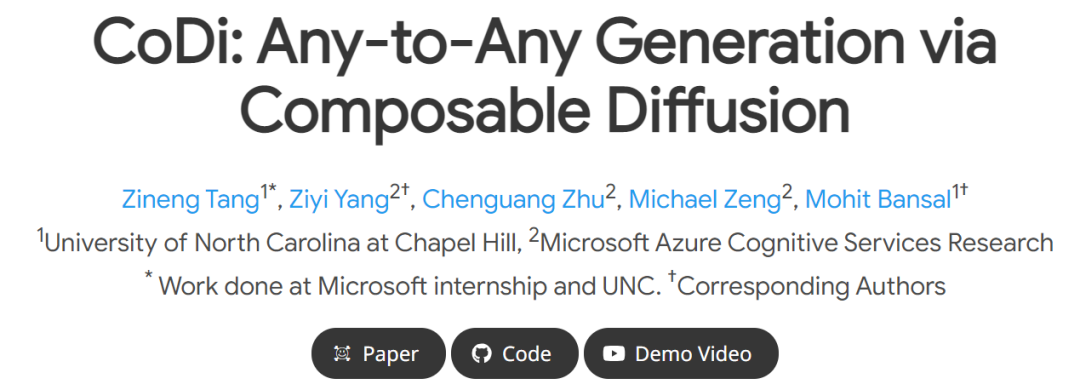14 038 0torch 如何实现两点分布采样，要求采100个样本，其中20个样本为数字1，80个为数字2

80 0DetectGPT:使用概率曲率的零样本机器生成文本检测
DetectGPT的目的是确定一段文本是否由特定的llm生成，例如GPT-3。为了对段落 x 进行分类，DetectGPT 首先使用通用的预训练模型（例如 T5）对段落 ~xi 生成较小的扰动。然后DetectGPT将原始样本x的对数概率与每个扰动样本~xi进行比较。如果平均对数比高，则样本可能来自源模型。
48 0【论文速递】WACV2022 - 基于小样本分割的多尺度Non-Novel片段消除方法
【论文速递】WACV2022 - 基于小样本分割的多尺度Non-Novel片段消除方法
93 0【论文速递】IJCV2022 - CRCNet:基于交叉参考和区域-全局条件网络的小样本分割
【论文速递】IJCV2022 - CRCNet:基于交叉参考和区域-全局条件网络的小样本分割
91 0【论文速递】PR2023 - 基于自正则原型网络的小样本语义分割
【论文速递】PR2023 - 基于自正则原型网络的小样本语义分割
94 0【图像隐藏】基于小波变换DWT实现数字盲水印嵌入提取含各类攻击附Matlab代码
【图像隐藏】基于小波变换DWT实现数字盲水印嵌入提取含各类攻击附Matlab代码
47 0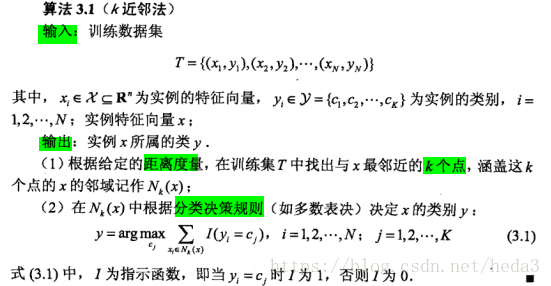K近邻算法（KNN）（包含手写体识别、约会类型识别的代码）

78 0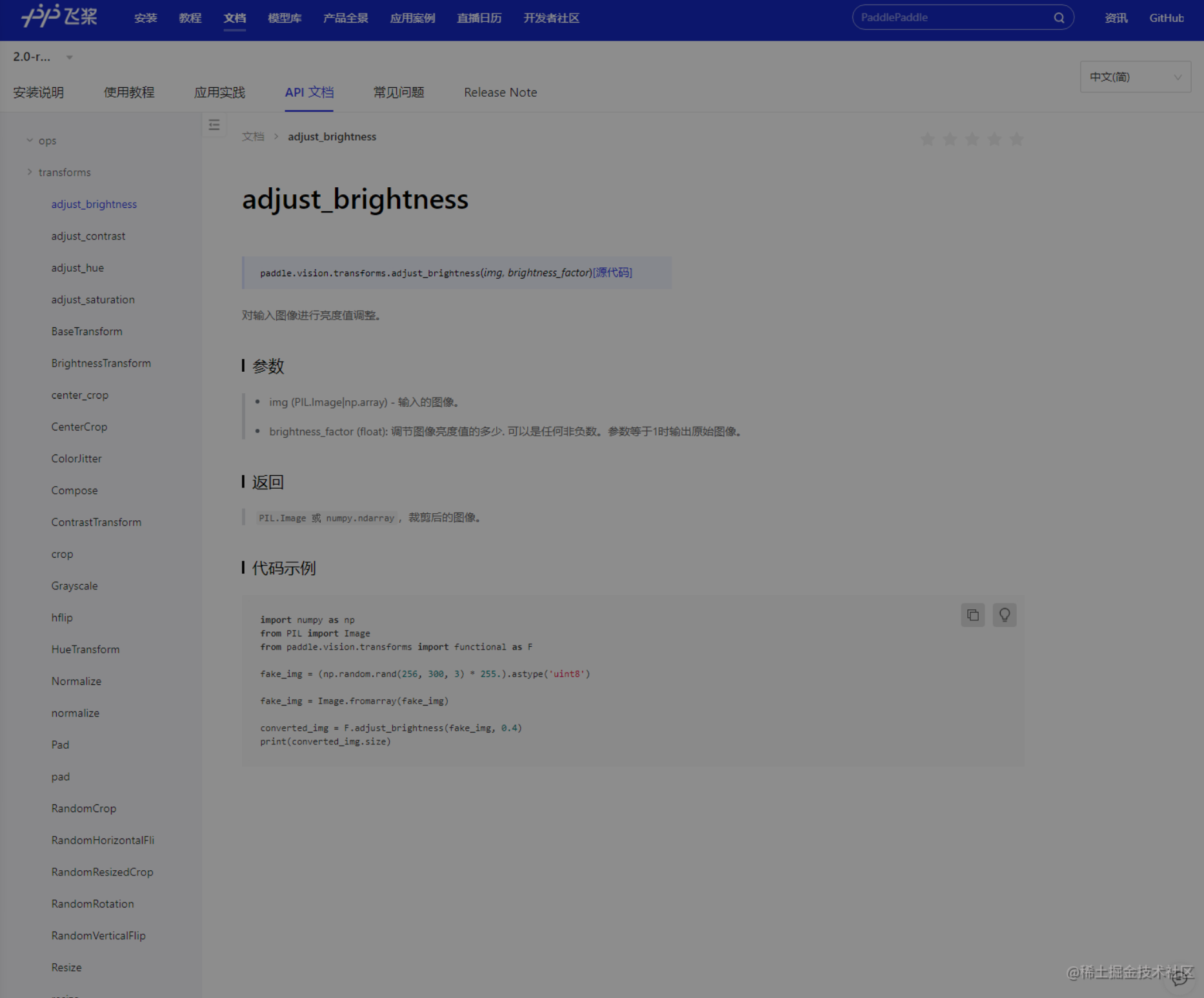27 0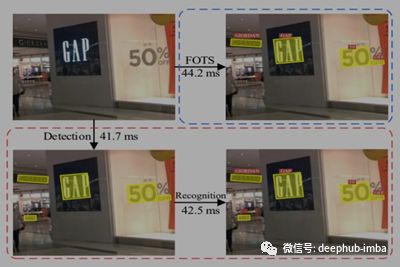FOTS：自然场景的文本检测与识别（上）
FOTS：自然场景的文本检测与识别
46 0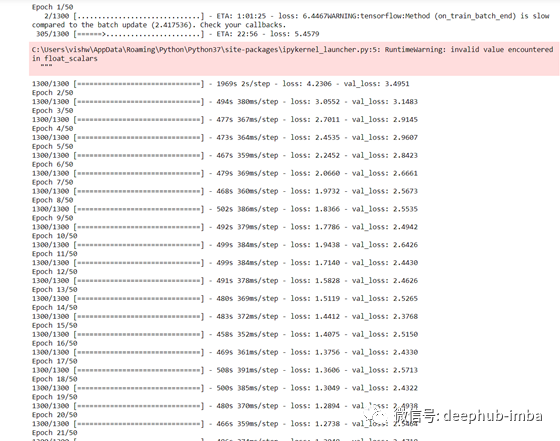FOTS：自然场景的文本检测与识别（下）
FOTS：自然场景的文本检测与识别
76 0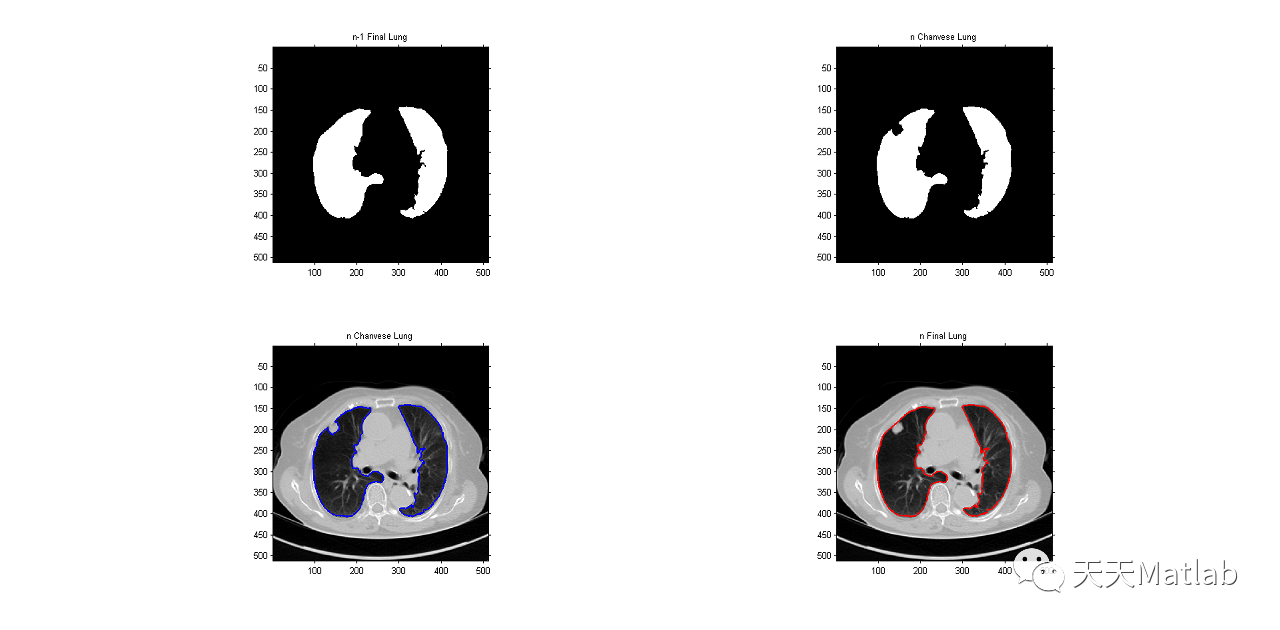【肺实质分割】基于主动轮廓模型和贝叶斯方法识别实现胸膜旁肺实质分割附matlab代码
【肺实质分割】基于主动轮廓模型和贝叶斯方法识别实现胸膜旁肺实质分割附matlab代码
60 0

【医学搜索Query相关性判断】赛题及baseline解读3153

140-弱监督机器学...1506573734.pdf